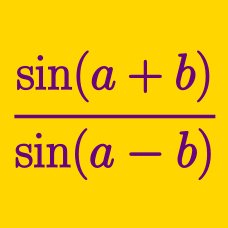Geometry

# Double Angle Identities

Given that $\sin^2 \theta = \frac{1}{3}$, what is the value of $72 \cos(2 \theta)$?

It is given that $\sin(\theta) = \frac{1}{\sqrt{37}}$. If $\cos ( 2 \theta ) = \frac{a}{b}$, where $a$ and $b$ are coprime positive integers, what is the value of $a+b$?

$x$ is an angle measured in radians and satisfies $\frac{-\pi}{2} < x < \frac{\pi}{2}$. It is given that $\sin(x) = \frac{8}{17}$. If $\sin(2x)$ can be written as $\frac{a}{b}$, where $a$ and $b$ are positive, coprime integers, what is the value of $a+b$?

The double angle identity states that

$\cos (2 \theta) = N \cos^2 \theta - M,$

where $N$ and $M$ are real numbers. What is the value of $N + M$?

The double angle identity states that

$\sin ( 2 \theta ) = N \sin \theta \cos \theta + M,$

where $N$ and $M$ are real numbers. What is the value of $N + M$?

×